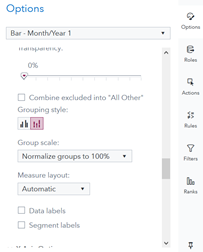## Formula calculation

Hello,
Does anyone knows the formula used to calculate ‘Normalise groups to 100%’ in the Group Scale which is found under Option in SAS Visual Analytics 8.5?

Thanks Catherine## Re: Formula calculation

Hello @cokello and welcome to the SAS Support Communities!

I have never used Visual Analytics, but from the documentation

If you select Normalize groups to 100%, then the values for each grouped bar are displayed as percentages of the total.

I conclude that you would divide the individual values (≥0) by the sum of all values (≥0) in the same group and multiply by 100% to obtain percentages (summing to 100% in each group).

In the example bar chart presented in the 2014 post Re: 100% Stacked Bar Charts:

If the revenues of the product lines A, B, C and D in facility region "South" are denoted by RA, RB, RC and RD, respectively, then the corresponding percentages pA, pB, etc. (depicted in the stacked bar chart as the heights of the blue, green, red and yellow section of the first bar) are calculated as

• pA = 100% ∙ RA/(RA+RB+RC+RD)
• pB = 100% ∙ RB/(RA+RB+RC+RD)
• and so on

and similarly for the other three regions.

Discussion stats